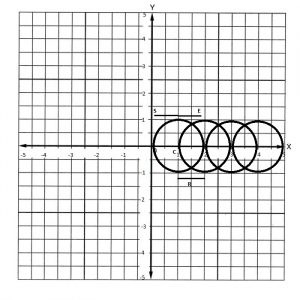# Minimum Circles needed to be removed so that all remaining circles are non intersecting

Given n Circles present in x-y plane such that all the circles have their center aligned on the x-axis.
The task is to remove some of them, such that no two circles are intersecting. Find the minimum number of circles that need to be removed.
Note : Touching circles are also considered to be intersecting.
Given N and an array of pair of integers. Each pair contains two integers c and r each, denoting the circle with radius r and center (c, 0).

Examples:

Input : N=4, arr={(1, 1), (2, 1), (3, 1), (4, 1)}
Output : 2Remove 2nd and 3rd circle to make the circles non-intersecting.

Input : N=4, arr={(1, 1), (4, 1), (5, 2), (7, 1)}
Output : 1

Approach:
Greedy strategy can be applied to solve the problem.

• Find staring and ending points of the diameter of the circles.
• Starting point would be equal to (c-r) and ending point would be equal to (c+r) where (c, 0) is the center of the particular circle and r is its radius.
• Sort the {start, end} pair according to the value of end point. Less the value of end point, less is its index.
• Start iterating the pairs and if the starting point of a circle is less then current end value, it means circles are intersecting hence increment the count. Else update the current end value.

Below is the implementation of the above approach:

 `// C++ implementation of the above approach ` `#include ` `#include ` `using` `namespace` `std; ` ` `  `struct` `circle { ` `    ``int` `start, end; ` `}; ` ` `  `// Comparison function modified ` `// according to the end value ` `bool` `comp(circle a, circle b) ` `{ ` `    ``if` `(a.end == b.end) ` `        ``return` `a.start < b.start; ` `    ``return` `a.end < b.end; ` `} ` ` `  `// Fucntion to return the count ` `// of non intersecting circles ` `void` `CountCircles(``int` `c[], ``int` `r[], ``int` `n) ` `{ ` `    ``// structure with start and ` `    ``// end of diameter of circles ` `    ``circle diameter[n]; ` ` `  `    ``for` `(``int` `i = 0; i < n; ++i) { ` `        ``diameter[i].start = c[i] - r[i]; ` ` `  `        ``diameter[i].end = c[i] + r[i]; ` `    ``} ` ` `  `    ``// sorting with smallest finish time first ` `    ``sort(diameter, diameter + n, comp); ` ` `  `    ``// count stores number of ` `    ``// circles to be removed ` `    ``int` `count = 0; ` ` `  `    ``// cur stores ending of first circle ` `    ``int` `cur = diameter.end; ` `    ``for` `(``int` `i = 1; i < n; ++i) { ` ` `  `        ``// non intersecting circles ` `        ``if` `(diameter[i].start > cur) { ` `            ``cur = diameter[i].end; ` `        ``} ` ` `  `        ``// intersecting circles ` `        ``else` `            ``count++; ` `    ``} ` ` `  `    ``cout << count << ``"\n"``; ` `} ` ` `  `// Driver Code ` `int` `main() ` `{ ` `    ``// centers of circles ` `    ``int` `c[] = { 1, 2, 3, 4 }; ` `    ``// radius of circles ` `    ``int` `r[] = { 1, 1, 1, 1 }; ` ` `  `    ``// number of circles ` `    ``int` `n = ``sizeof``(c) / ``sizeof``(``int``); ` ` `  `    ``CountCircles(c, r, n); ` ` `  `    ``return` `0; ` `} `

Output:

```2
```

Time Complexity: O(N*log(N))
where N is the number of circles.

My Personal Notes arrow_drop_upCheck out this Author's contributed articles.

If you like GeeksforGeeks and would like to contribute, you can also write an article using contribute.geeksforgeeks.org or mail your article to contribute@geeksforgeeks.org. See your article appearing on the GeeksforGeeks main page and help other Geeks.

Please Improve this article if you find anything incorrect by clicking on the "Improve Article" button below.

Article Tags :
Practice Tags :

Be the First to upvote.

Please write to us at contribute@geeksforgeeks.org to report any issue with the above content.